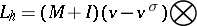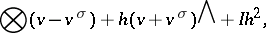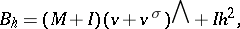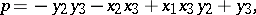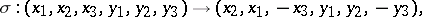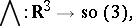# Kowalewski top

Kovalevskaya top

In 1889, S. Kovalevskaya [a11] solved the following problem: find all rigid bodies, rotating about a fixed point in the presence of gravity, such that the equations of motion are integrable in the sense of Kowalewski. The latter means that the system admits solutions, expressible as Laurent series in time, which contain a number of free parameters equal to the number of degrees of freedom minus one; the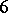-dimensional phase space for such a rigid body is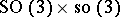. After the standard reduction to the body coordinates (reduction to the Lie algebra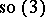), for the system to be integrable in the sense of Kowalewski, the Jacobian matrix of the indicial locus (of the equations of motion) must have integer spectrum. This strong condition leads to the following three cases of integrable rotating bodies: i) the Euler top, for which the fixed point and the centre of mass coincide; ii) the Lagrange top, which is an axially-symmetric rigid body, with centre of mass on the axis of symmetry; and iii) the so-called Kowalewski top, which is a solid body rotating about a fixed point such that:

a) its principal moments of inertia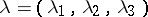with regard to the fixed point satisfy the relation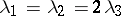;

b) its centre of mass belongs to the equatorial plane (corresponding to the momentand) through the fixed point. The motion (in body coordinates) is governed by the equations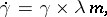where,anddenote, respectively, the angular momentum, the centre of mass and the unit vector in the direction of gravity; also,. Upon appropriate rescaling and picking the axes of inertia in the equatorial plane, one can achieve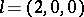and. Besides the Casimir invariants, the angular momentum in the direction of gravity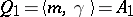, the length of the squared gravity axis, and the conservation of energy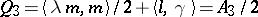, Kovalevskaya exhibited ath invariant, which is quartic, upon giving weightto the's andto the's: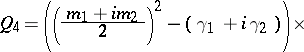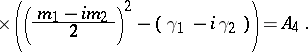## Algebraic integrability.

The motion is "algebraically integrable" in a sense defined by M. Adler and P. van Moerbeke [a3], [a5]: for fixed but arbitrary values of the constants of motion, the level surfaces are affine partsof Abelian varieties(complex algebraic tori; cf. Algebraic torus). For the Kowalewski top, the level surfacesare affine partsof Abelian surfacesof polarization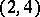(cf. Polarized algebraic variety), and the complement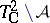is a union of two ample divisors (curves) of genus, each of them being a doubly ramified (inpoints) cover of an elliptic curve. The Kowalewski system then "linearizes" on these Abelian varieties, and the motion can be written in terms of Abelian integrals (quadratures; cf. also Abelian integral), involving an intricate change of variables, governed by the algebraic geometry above; see [a13] and for the quadratures [a9]. A birational mapping transforms Kowalewski's motion into a geodesic flow onfor the Manakov metric [a4].

## Lax pairs.

The Kowalewski top equations can be expressed as a Lax pair (see [a8]) of the following form: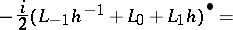(a1)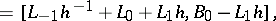whereis an indeterminate, and where the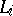and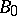arematrices, defined below. The motion then linearizes on the Jacobian of the corresponding spectral curve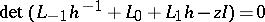, which is a double cover (of genus) of a hyper-elliptic curve of genus, ramified atpoints. The matrices in (a1) are given by: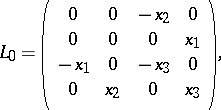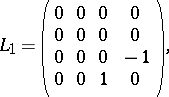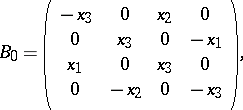where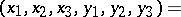Such Lax pairs arise in the context of Kostant–Kirillov–Souriau orbits, for Lie algebra splittings into two Lie subalgebras, as summarized by the Adler–Kostant–Symes theorem [a1], [a2]. A variation of the latter theorem was applied in [a8] to a loop algebra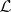(cf. also Loop) with a new bracket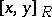, constructed as follows: consider the Lie algebraand its decomposition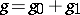into even and odd subspaces for the automorphism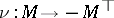. Then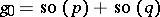. From this, construct the twisted loop algebra, together with its splitting and dual splitting, for a non-degenerate ad-invariant pairing: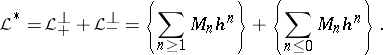For the Kowalewski top, pick, viewed as a-dimensional subspace of the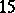-dimensional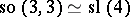. After conjugation by a constant matrix, the Lax pair has the form (a1).

Another Lax pair mentioned in [a4] and, in a slight variation, in [a12] is based on the following idea: the birational mapping, mentioned above, from the Kowalewski top to the geodesic flow onfor the Manakov metric leads to the following Lax pair: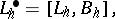(a2)

and the motion linearizes on the Jacobian of the spectral curve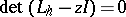, which is Kowalewski's original hyper-elliptic curve. In (a2),